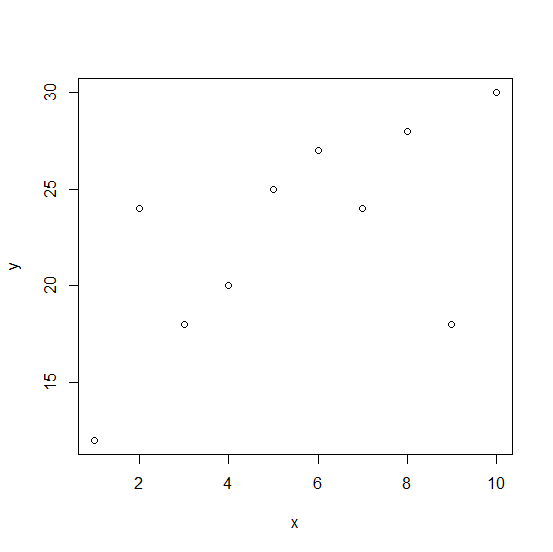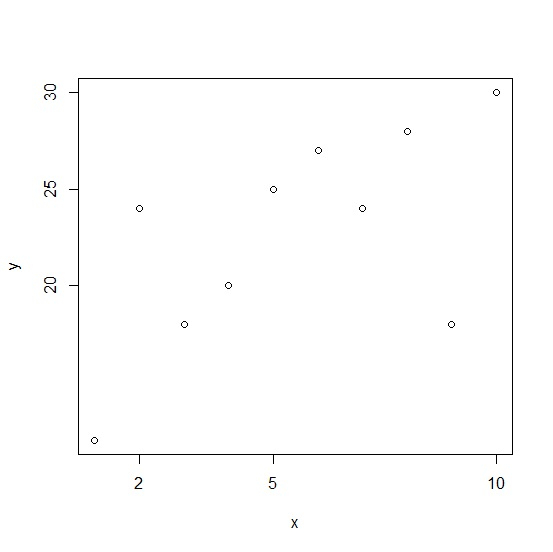# How to change the axes labels using plot function in R?

In a plot, the axes labels help us to understand the range of the variables for which the plot is created. While creating a plot in R using plot function, the axes labels are automatically chosen but we can change them. To do this, firstly we have to remove the axes then add each of the axes with the labels we want and then create the box for the plot.

## Example

Consider the below data −

> x<-1:10
> y<-c(12,24,18,20,25,27,24,28,18,30)

Creating the scatterplot between x and y −

> plot(x,y)

## OutputChanging the axes labels for X and Y axes −

> plot(x,y,axes=FALSE)+
+ axis(side = 1, at = c(2,5,10))+
+ axis(side = 2, at = c(20,25,30))+
+ box()

## Output